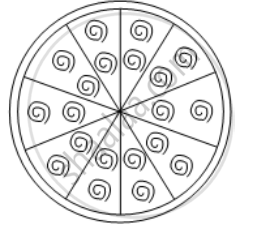# A brooch is made with silver wire in the form of a circle with diameter 35 mm. The wire also used in making 5 diameters which divide the circle into 10 equal sectors as shown in Fig. Find - Mathematics

Sum

A brooch is made with silver wire in the form of a circle with diameter 35 mm. The wire also used in making 5 diameters which divide the circle into 10 equal sectors as shown in Fig. Find:

(i) the total length of the silver wire required

(ii) the area of each sector of the brooch

#### Solution

(i) We have,Total length of the silver wire = Circumference of the circle of radius

35/2 mm + Length of five diameters

= 2π × \frac { 35 }{ 2 } + 5 × 35 mm

=( 2\times \frac{22}{7}\times \frac{35}{2}+175)\text{ mm}

= 285 mm

(ii) The circle is divided into 10 equal sectors, Therefore, Area of each sector of the brooch

= 1/10 (Area of the circle)

= 1/10 × π × (35/2)^2 cm^2

\text{=}\frac{1}{10}\times \frac{22}{7}\times \frac{35}{2}\times\frac{35}{2}mm^{2}

=\frac{385}{4}mm^{2}

Concept: Circumference of a Circle
Is there an error in this question or solution?